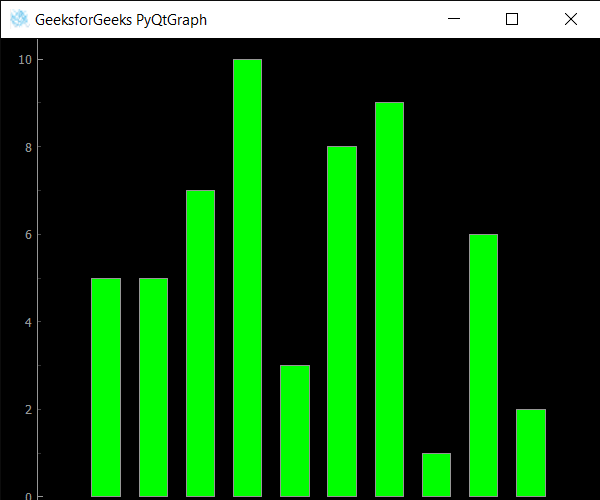# PyQtGraph – Getting Tool Tip Duration of Plot Window

• Last Updated : 25 Sep, 2020

In this article we will see how we can get tool tip duration of the plot window in the PyQtGraph module. PyQtGraph is a graphics and user interface library for Python that provides functionality commonly required in designing and science applications. Its primary goals are to provide fast, interactive graphics for displaying data (plots, video, etc.) and second is to provide tools to aid in rapid application development (for example, property trees such as used in Qt Designer).Plot windows consist of two main parts: the Plot Panel containing the actual plotted graphics and the Control Panel. The tooltip, infotip, or hint is a common graphical user interface element displayed as an informational text box when hovering over an item. The user hovers the pointer over an item, without clicking it, and a tooltip may appear a small “hover box” with information about the item being hovered over. It can be set with the help of `setToolTip` method. Tool tip duration is the time period for which tip will appear after the duration it will get disappeared. It can be set with the help of `setToolTipDuration` method.

We can create a plot window with the help of command given below

Attention geek! Strengthen your foundations with the Python Programming Foundation Course and learn the basics.

To begin with, your interview preparations Enhance your Data Structures concepts with the Python DS Course. And to begin with your Machine Learning Journey, join the Machine Learning - Basic Level Course

```# creating a pyqtgraph plot window
window = pg.plot()
```

In order to do this we use `toolTipDuration` method with the plot window object

Syntax : window.toolTipDuration()

Argument : It takes no argument

Return : It returns integer

Below is the implementation

 `# importing QtGui to use QIcon``from` `PyQt5.QtGui ``import` `*` ` ` `# importing pyqtgraph as pg``import` `pyqtgraph as pg`` ` `# importing QtCore and QtGui from the pyqtgraph module``from` `pyqtgraph.Qt ``import` `QtCore, QtGui`` ` `# importing numpy as np``import` `numpy as np`` ` `import` `time`` ` `# creating a pyqtgraph plot window``window ``=` `pg.plot()`` ` `# icon for plot window``icon ``=` `QIcon(``"logo.png"``)`` ` `# setting icon to the plot window``window.setWindowIcon(icon)`` ` `# setting window geometry``# left = 100, top = 100``# width = 600, height = 500``window.setGeometry(``100``, ``100``, ``600``, ``500``)`` ` `# title for the plot window``title ``=` `"GeeksforGeeks PyQtGraph"`` ` `# setting window title to plot window``window.setWindowTitle(title)`` ` `# create list for y-axis``y1 ``=` `[``5``, ``5``, ``7``, ``10``, ``3``, ``8``, ``9``, ``1``, ``6``, ``2``]`` ` `# create horizontal list i.e x-axis``x ``=` `[``1``, ``2``, ``3``, ``4``, ``5``, ``6``, ``7``, ``8``, ``9``, ``10``]`` ` `# create pyqt5graph bar graph item ``# with width = 0.6``# with bar colors = green``bargraph1 ``=` `pg.BarGraphItem(x ``=` `x, height ``=` `y1, width ``=` `0.6``, brush ``=``'g'``)`` ` `# add item to plot window``# adding bargraph item to the window``window.addItem(bargraph1)`` ` `# text for tool tip``text ``=` `"This is Bar Graph"`` ` `# setting window tool tip``window.setToolTip(text)`` ` `# setting toop tip duration = 2seconds``window.setToolTipDuration(``2000``)`` ` `# getting tool tip duration of plot window``value ``=` `window.toolTipDuration()`` ` `# printing the value``print``(``"Tool Tip Duration : "` `+` `str``(value))`` ` `# main method``if` `__name__ ``=``=` `'__main__'``:``     ` `    ``# importing system``    ``import` `sys``     ` `    ``# Start Qt event loop unless running in interactive mode or using ``    ``if` `(sys.flags.interactive !``=` `1``) ``or` `not` `hasattr``(QtCore, ``'PYQT_VERSION'``):``        ``QtGui.QApplication.instance().exec_()``        `

Output :```Tool Tip Duration : 2000
```

My Personal Notes arrow_drop_up